# Preprocessing and analysis of spike and local field potential data

## Introduction

In this tutorial, we will show how to compute the ongoing phase and power of the LFP at the time of neuronal firing, and statistics of spike-LFP phase-coupling, such as the Rayleigh probability or the PPC (Vinck et al., 2010, NeuroImage, 2011, J Comp Neurosci). To this end, we will use a dataset from Pascal Fries and colleagues (2001, 2008), in which both LFPs and spikes were recorded simultaneously. In this experiment, V4 LFP and spike data was recorded while monkeys performed a visual attention task. A moving stimulus grating was presented in the recorded neurons’ receptive fields, and another stimulus was presented outside the recorded neurons’ receptive fields. In a given trial, the grating stimulus in the receptive field was either attended (attention inside the receptive field) or not attended (distractor; attention outside the receptive field). In this tutorial, we analyze the spike data around the presentation of the stimulus until the time of the first stimulus change (of either distractor or target).

## Background

A popular choice of signals to study rhythmic neuronal synchronization is the combination of spiking activity and the LFP (Local Field Potential). Spike-LFP measures are more likely to detect phase synchronization than spike-spike measures, because the LFP measures the compound synaptic activity generated by a large pool of neurons. This sensitivity is especially advantageous for the study of long-range interactions. Many studies have revealed functionally relevant changes in cross-areal spike-LFP phase synchronization that would have been difficult to detect using measures of spike-spike synchronization (Pesaran et al., 2008, Liebe et al., 2012, Womelsdorf et al., 2007, Siapas et al., 2005, Gregoriou et al., 2009, Benchenane et al., 2010, Colgin et al., 2009, Sigurdsson et al., 2010). At the same time, spike-LFP measures avoid the problem that volume-conduction and common referencing can create spurious correlations between LFP signals.

In this tutorial we will use the approach to compute a single phase per individual spike (see Figure below) and a consistency measure over the set of spike phases, which is the conventional technique in the hippocampus field (e.g., see O’Keefe et al., 1993; Bragin et al., 1995, Csicsvari et al., 1999) and which was recently applied by us to visual cortex data (Vinck et al., 2010, J Neurosci, Womelsdorf et al., 2012, PNAS). This approach has two advantages. Firstly, by centering a segment of the LFP around each individual spike, we ensure that the phase of the LFP is determined locally, as opposed to directly computing (spike-field) coherence between spike and LFP signals, where spikes fall at arbitrary positions relative to the LFP segments. Empirical data shows that this is an important consideration, because spike-triggered LFP averages show only few side lobes (Fries et al., 2001). Secondly, we can directly compare the statistics of the spike-triggered LFP spectrum to the spike-triggered average, i.e. we can compare the frequency-domain representation of the LFP around the spikes with its time-domain representation, which may offer complementary information. Thirdly, by measuring a single phase per individual spike we can explicitly control the bias as a function of the number of spikes (Vinck et al., 2010, 2011), which is a serious problem for spike-field coherence measures (Zeitler et al., 2006, Lepage et al., 2011, Vinck et al., 2011, J Comp Neurosci). For further details on the comparison between these different measures and their advantages/disadvantages, we refer to Vinck et al. (2011).## Procedure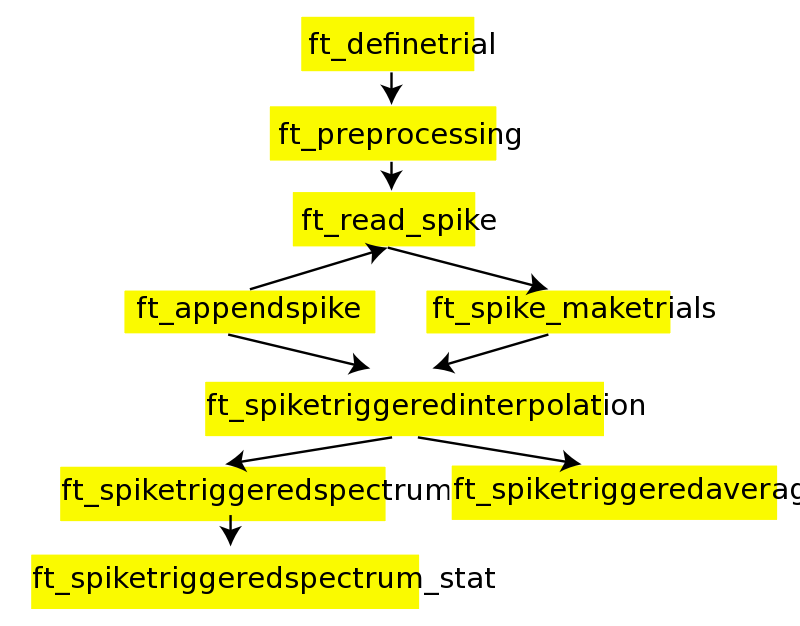### Preprocessing

The data for this tutorial can be downloaded here. Make sure you add the main FieldTrip directory to your path and run ft_defaults. We first read in the spike data by ft_read_spike and select the following channels for analysis from the spike structure using ft_spike_select by

``````filename         = 'p029_sort_final_01.nex';

cfg              = [];
cfg.spikechannel = {'sig002a_wf', 'sig003a_wf'};
spike            = ft_spike_select(cfg, spike);
``````

giving a spike structure

``````spike =

label: {'sig002a_wf'  'sig003a_wf'}
timestamp: {[1x164456 int32]  [1x134803 int32]}
waveform: {[1x32x164456 double]  [1x32x134803 double]}
unit: {[1x164456 double]  [1x134803 double]}
hdr: [1x1 struct]
cfg: [1x1 struct]
``````

For more information on the spike format see the spike tutorial. Briefly, the field spike.timestamps contains the times of spiking for every cell in the unit of the recording system (called ‘timestamps’).

We then construct a cfg.trl matrix to preprocess the LFP data. In this case, the unit of cfg.trl should be samples (not timestamps, as with ft_spike_maketrials), by

``````function trl = trialfun_stimon_samples(cfg)
correctresponse  = 10041;
begintrial       = 10044;
endtrial         = 10045;
stimon           = 10030;
distractorChange = 12000;
targetChange     = 12001;
attCnds          = 20001:20004; % att in/out by target change first/second
E          = struct2cell(event);
sel        = ~cellfun('isempty', E(2,:)); % events with empty values should not be considered
samples    = cell2mat(E(1,sel)); % now in vector form
value      = cell2mat(E(2,sel));
begmark    = find(value==begintrial); % loop through the trial beginnings
endmark    = find(value==endtrial); % loop through the trial beginnings
trl        = []; % initialize the cfg.trl
for k=1:length(begmark)
vals = value(begmark(k):endmark(k));
if any(ismember(vals,attCnds)) && ~isempty(find(vals==correctresponse))
% create the trl matrix in sample units
samp = samples(begmark(k):endmark(k)); % in timestamp units
beginSamp      = samp(find(vals==stimon));
sampDistractor = samp(find(vals==distractorChange));
sampTarget     = samp(find(vals==targetChange));
endSamp        = min([sampTarget(:);sampDistractor(:)]); % limit until first change
offset         = -round(hdr.Fs*2.75);
trl            = [trl; [beginSamp+offset endSamp offset]];
end
end
``````

Subsequently, we read out the LFP data using

``````% get the cfg.trl
cfg          = [];
cfg.dataset  = filename;
cfg.trialfun = 'trialfun_stimon_samples';
cfg          = ft_definetrial(cfg);

% read in the data in trials
cfg.padding   = 10; % length to which we pad for filtering
cfg.dftfreq   = [60-1*(1/10):(1/10):60+1*(1/10) ]; % filter out 60 hz line noise
cfg.dftfilter = 'yes';
data_lfp      = ft_preprocessing(cfg); % read in the LFP
``````

The LFP data is now represented in a structure that has the following standard form (see ft_datatype_raw

``````data_lfp =
hdr: [1x1 struct]
label: {4x1 cell}
time: {1x600 cell}
trial: {1x600 cell}
fsample: 1000
sampleinfo: [600x2 double]
cfg: [1x1 struct]
``````

Here, every cell of data_lfp.trial contains a chan x time data matrix for one trial (# trials = 600).

It is important to note that we assume that there are no gaps in the recording, i.e. that the LFP recording is continuous. It may occasionally occur that (at least for Neuralynx software this is known) there are gaps in the LFP recording because the recording software has been turned on and off, such that there is one Ncs file with a large gap. In that case, one must take care of linking timestamps and samples as there will not be a linear relationship anymore. We refer to this page for potential solutions.

The critical pieces of information needed to link LFPs to spikes are the number of timestamps per LFP sample, the LFP sampling rate and the first timestamp of the recording. If one reads out the LFP files using ft_read_spike then this information is represented in data.hdr.Fs, data.hdr.TimeStampPerSample and data.hdr.FirstTimeStamp. In this case,

``````data_lfp.hdr =
Fs: 1000
nSamples: 7555983
FirstTimeStamp: 0
TimeStampPerSample: 40
``````

The relationship between (unrounded) LFP sample and a timestamp ts (of a spike) is then given as

``````sample = double(ts-FirstTimeStamp) / double(TimeStampPerSample) + 1;
``````

The factor +1 arises because the first LFP sample is numbered 1, not 0.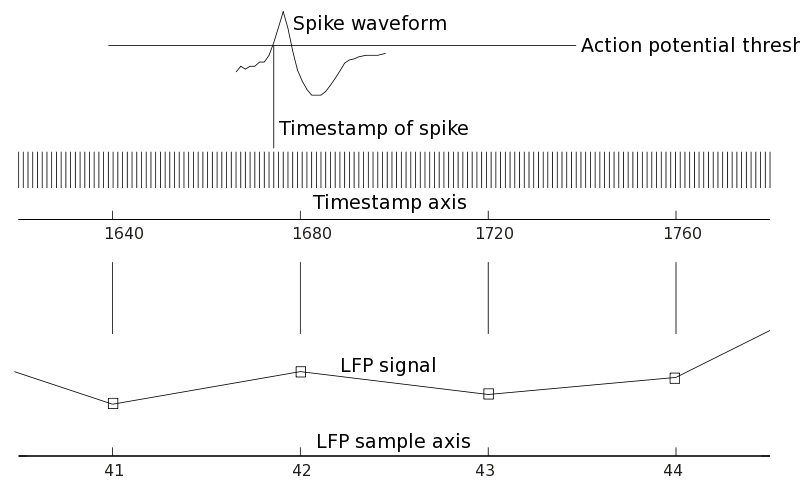We now have two options to further process the raw spike data such that the resulting spike structure has the same trial definition as the data_lfp structure. First of all, we can directly create trials for the spike structure, by

``````cfg           = [];
cfg.dataset   = filename;
cfg.trialfun  = 'trialfun_stimon_samples';
cfg           = ft_definetrial(cfg);
trl           = cfg.trl;

cfg           = [];
cfg.hdr       = data_lfp.hdr; % contains information for conversion of samples to timestamps
cfg.trlunit   = 'samples';
cfg.trl       = trl; % now in samples
spikeTrials   = ft_spike_maketrials(cfg,spike);
``````

giving a struct

``````spikeTrials =
label: {'sig002a_wf'  'sig003a_wf'}
timestamp: {[1x83601 int32]  [1x61513 int32]}
waveform: {[1x32x83601 double]  [1x32x61513 double]}
unit: {[1x83601 double]  [1x61513 double]}
hdr: [1x1 struct]
cfg: [1x1 struct]
time: {[1x83601 double]  [1x61513 double]}
trial: {[1x83601 double]  [1x61513 double]}
trialtime: [600x2 double]
``````

where spike.trial{i} and spike.time{i} specify, for every i-th unit, the trial in which the spike was fired and the time at which it was fired relative to the trigger, respectively.

An equivalent method (but potentially more error-prone!) would have been to directly use the timestamp representation per event to create the trials, i.e. use the ‘trialfun_stimon’ that we defined in the spike tutorial. For the purpose of walking through this tutorial, you should copy and paste the code of trialfun_stimon.m (from the spike tutorial) in the MATLAB editor and save the m-file as `trialfun_stimon.m`. Alternatively you can download the trial function trialfun_stimon.m.

``````cfg          = [];
cfg.dataset  = filename;
cfg.trialfun = 'trialfun_stimon'; % this was defined in the spike tutorial
cfg          = ft_definetrial(cfg);
cfg.timestampspersecond = 40000;
spikeTrials2 = ft_spike_maketrials(cfg,spike);
``````

A second method would have been to append the spikes to the LFP data in binary data format, by

``````data_all = ft_appendspike([],data_lfp, spike);
``````

We then get

``````data_all =
hdr: [1x1 struct]
label: {6x1 cell}
time: {1x600 cell}
trial: {1x600 cell}
fsample: 1000
sampleinfo: [600x2 double]
cfg: [1x1 struct]
``````

A disadvantage of the second method relative to the first methods is that the spike times are converted to samples, such that we introduce a (minor) distortion of the estimated spike-LFP phases. In practice, this has only minor consequences if data_all.fsample is high and the frequency of interest at which we want to measure the spike-LFP phase is low. However, for quantifying locking at frequencies of >100 Hz, this error may substantially reduce locking values. Two further disadvantages are that it unnecessarily decreases the memory-load, and makes it more difficult to link the spike-LFP phase information to information that was stored per individual spike, e.g., the AP waveforms.

The spike trains have now been binarized (as mentioned earlier, values higher than 1 can occasionally occur if the sample frequency is not high enough), and a piece of data.trial{i} has the following content:

``````disp(data_all.trial{1}(:,4002:4005))
-12.3496  -12.9317  -16.8991  -14.4778 % the LFP chans
-3.5391    3.5410    2.4398    0.0795
4.3475    9.5496    4.8793    3.0704
14.5906    9.6772    7.5005   11.9295
0         0         0         0 % the binarized spike trains
0    1.0000         0         0
``````

### Analyzing spikes and LFPs recorded from the same electrode

To analyze high-frequency phase-coupling between spikes and LFPs recorded from the same electrode, it is important to consider that the spike’s action potential has considerable signal energy even below 100 Hz. As a consequence, spikes are strongly locked to the high-frequency (same-electrode) LFP components. To solve this issue, we need to discard the portion of the LFP around the occurrence of the spikes. This is performed by the function ft_spiketriggeredinterpolation. The discarded portion of the LFP is then replaced by NaNs (not a number) or interpolated based on the remaining LFP data. The output is a raw data structure again that can serve as input to ft_spiketriggeredspectrum and ft_spiketriggeredaverage.

``````cfg              = [];
cfg.method       = 'nan'; % replace the removed segment with nans
cfg.timwin       = [-0.002 0.002]; % remove 4 ms around every spike
cfg.spikechannel = spike.label{1};
cfg.channel      = data_lfp.label(2);
data_nan         = ft_spiketriggeredinterpolation(cfg, data_all);

cfg.method       = 'linear'; % remove the replaced segment with interpolation
data_i           = ft_spiketriggeredinterpolation(cfg, data_all);
``````

We illustrate this method by plotting the data:

``````figure,
plot(data_i.time{1},data_i.trial{1}(2,:),'g-'), hold on, plot(data_i.time{1}, data_i.trial{1}(5,:),'r')
hold on
plot(data_nan.time{1},data_nan.trial{1}(2,:),'go')
hold on
plot(data_all.time{1},data_all.trial{1}(2,:),'k-')
xlim([0.9 1])
xlabel('time (s)')
``````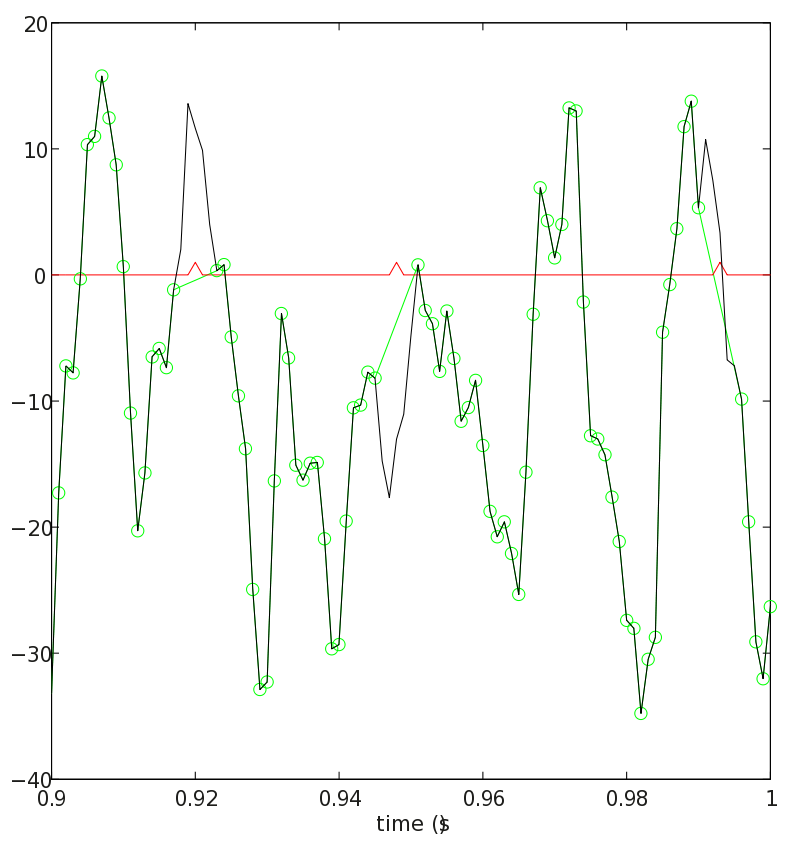### Computing the spike triggered average LFP

The first step in the analysis of spike-LFP phase-coupling should be the computation of the spike-triggered average (STA) of the LFP. This is the time-domain counterpart of the spike-triggered spectrum of the LFP (ft_spiketriggeredaverage). The time-domain representation of the spike-triggered LFP may reveal features that are not easily understood from the frequency-domain representation, e.g., whether there are oscillatory cycles at some frequency, a characteristic main lobe, and leakage of the spike waveform into the LFP. To compute the STA we perform:

``````cfg              = [];
cfg.timwin       = [-0.25 0.25]; % take 400 ms
cfg.spikechannel = spike.label{1}; % first unit
cfg.channel      = data_lfp.label(1:4); % first four chans
cfg.latency      = [0.3 10];
staPost          = ft_spiketriggeredaverage(cfg, data_all);

% plot the sta
figure
plot(staPost.time, staPost.avg(:,:)')
legend(data_lfp.label)
xlabel('time (s)')
xlim(cfg.timwin)
``````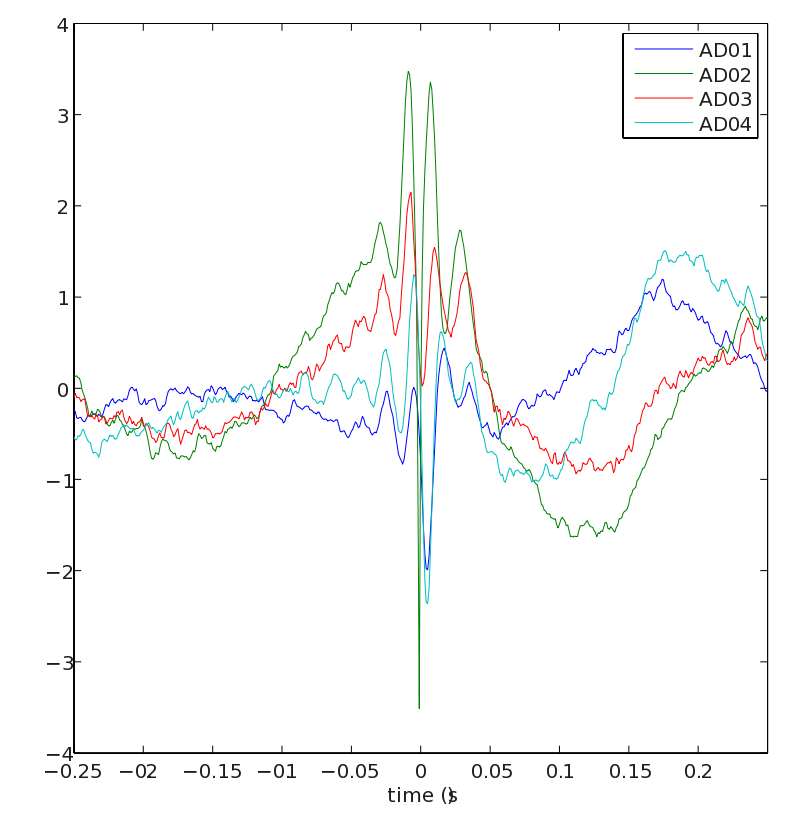The STA reveals several oscillatory cycles at gamma frequency around the spike. For channel ‘AD02’, we see a characteristic sharp peak around t = 0, caused by the occurrence of the spike itself.

We also show the STA for the pre-stimulus period:

``````cfg              = [];
cfg.timwin       = [-0.25 0.25]; % take 400 ms
cfg.spikechannel = spike.label{1}; % first unit
cfg.channel      = data_lfp.label(1:4); % first four chans
cfg.latency      = [-2.75 0];
staPre           = ft_spiketriggeredaverage(cfg, data_all);

figure
plot(staPre.time, staPre.avg(:,:)')
legend(data_lfp.label)
xlabel('time (s)')
xlim(cfg.timwin)
``````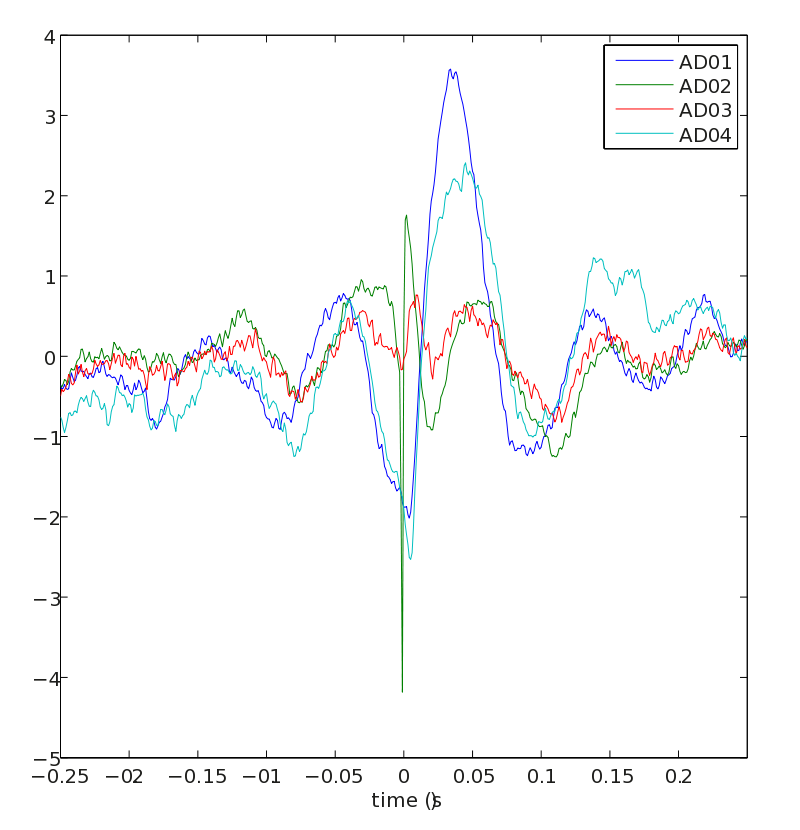The pre-stimulus STA reveals locking of spikes to alpha LFP cycles.

### Computing the phases of spikes relative to the ongoing LFP

After we obtained, from the preprocessing steps, a data structure containing the spike information that was either appended in binarized form to the LFP data (through ft_appendspike) or stored in a separate spike structure (through ft_spike_maketrials) we can proceed with computing the phase of the LFP relative to single spikes. It is also possible (not covered in this tutorial) to analyze the data_all structure (containing both LFP and spike data) using ft_freqanalysis and subsequently compute connectivity measures with ft_connectivityanalysis. This would have been the method to compute the spike-field coherence metric. However, this latter methodology has disadvantages, as explained in the introduction.

The idea of our procedure is to take an LFP segment around the spike and compute the Discrete Fourier Transform of that. Two algorithms are available for computing the phases of single spikes relative to the LFP. The first algorithm ft_spiketriggeredspectrum_fft computes the FFT locally around every spike by calling MATLAB’s FFT function and uses the same window length for all frequencies. The other algorithm in ft_spiketriggeredspectrum_convol computes the phase for every frequency separately by computing the DFT for a given frequency through convolution. Different time-windows per frequency are then allowed. The choice of the algorithm at the user-end is determined by calling ft_spiketriggeredspectrum with cfg.method = ‘mtmfft’ or cfg.method = ‘mtmconvol’.

The MTMFFT algorithm allows that only one spikechannel can be selected at a time. One can either have the spike train in binarized format or enter it separately as a third input.

``````cfg              = [];
cfg.method       = 'mtmfft';
cfg.foilim       = [20 100]; % cfg.timwin determines spacing
cfg.timwin       = [-0.05 0.05]; % time window of 100 msec
cfg.taper        = 'hanning';
cfg.spikechannel = spike.label{1};
cfg.channel      = data_lfp.label;
stsFFT           = ft_spiketriggeredspectrum(cfg, data_all);
``````

We then obtain

``````stsFFT =
lfplabel: {4x1 cell}
freq: [20 30 40 50 60 70 80 90 100]
dimord: '{chan}_spike_lfpchan_freq'
fourierspctrm: {[83616x4x9 double]}
time: {[83616x1 double]}
trial: {[83616x1 double]}
trialtime: [600x2 double]
label: {'sig002a_wf'}
cfg: [1x1 struct]
``````

This structure is a structure formatted as ft_datatype_spike with the additional field sts.fourierspctrm. For every i-th unit, the field stsFFT.fourierspctrm{i} contains complex valued LFP fourierspectra taken around the occurrence of every spike. The spike phases for the unit ‘sig001U_wf’ are thus obtained by

``````ang = angle(stsFFT.fourierspctrm{1})
``````

and the magnitude of the LFP is obtained by

``````mag = abs(stsFFT.fourierspctrm{1})
``````

The convolution algorithm (cfg.method = ‘mtmconvol’) accepts spikes both in binarized (raw) and spike format. Multiple spike channels can be selected at the same time. For every frequency, we can specify a different time window as well, such that for example the number of cycles per frequency can be kept constant. The algorithm allows for large speed-ups if the number of spikes and units is large, as the instantaneous LFP phase is determined (only once) for all possible time-points through convolution. However this method is not preferred if there are few spikes and very long LFP traces (in this case iteratively using the FFT method would be faster).

``````cfg           = [];
cfg.method    = 'mtmconvol';
cfg.foi       = 20:10:100;
cfg.t_ftimwin = 5./cfg.foi; % 5 cycles per frequency
cfg.taper     = 'hanning';
stsConvol     = ft_spiketriggeredspectrum(cfg, data_all);
``````

Note that we could have also used a third spike input instead of the data_all input.

``````stsConvol2    = ft_spiketriggeredspectrum(cfg, data_lfp, spikeTrials);
``````

The latter way of calling ft_spiketriggeredspectrum is advantageous because 1) it is more memory efficient, and 2) within ft_spiketriggeredspectrum_convol, the spike samples do not have to be converted back to spike times. Instead, the spike times are exact and readily available, such that the phase estimation is more accurate as the raw spike time is used to determine the spike-LFP phase, instead of the rounded spike sample that is obtained using ft_appendspike. This is relevant when studying fast LFP oscillations.

The output from the mtmconvol method is:

``````stsConvol =
lfplabel: {4x1 cell}
freq: [20 30.1205 40.3226 50 60.9756 71.4286 80.6452 89.2857 100]
label: {'sig002a_wf'  'sig003a_wf'}
fourierspctrm: {[83616x4x9 double]  [61526x4x9 double]}
time: {[83616x1 double]  [61526x1 double]}
trial: {[83616x1 double]  [61526x1 double]}
dimord: '{chan}_spike_lfpchan_freq'
trialtime: [600x2 double]
cfg: [1x1 struct]
``````

Note that in this instance data is present for multiple units.

### Computing statistics on the output from ft_spiketriggeredspectrum.m

Statistics on the obtained data are computed using the function ft_spiketriggeredspectrum_stat. The configuration cfg.method tells us which statistic to compute. These include standard Rayleigh test, mean phase, and the Pairwise Phase Consistency metrics (Vinck et al., 2010, Neuroimage, Vinck et al., 2011, J Comput Neurosci). Three versions of the PPC measure are available: ‘ppc0’, ‘ppc1’, and ‘ppc2’. The ‘ppc0’ measure considers all pairs of spikes across all trials, is not biased by spike count (as the phase locking value is) and is fastest to compute, however can be influenced by history effects within the spike trains (e.g., refractoriness and bursting). This is not an issue however if the number of trials is large, as it is here. The ‘ppc1’ measure avoids this problem of history effects within spike trains, and the ‘ppc2’ measure improves on the ‘ppc1’ measure by being robust against dependencies between spike phase and spike rate (as with gamma phase shifting, Vinck et al. (2010, J Neurosci)), at the cost of an increase in variance.

The configuration cfg.timwin determines whether we compute the statistics in a time-resolved way (e.g., by cfg.timwin = 0.5, taking sliding windows of 500 ms) or in a non-time resolved way, i.e. across all spikes available in a certain cfg.latency window. We compute our locking statistics only w.r.t. to the LFP channels that were not recorded from the same electrode as the unit under consideration, to avoid bleeding of the unit’s spike waveform energy into the LFP. We then average the spike phases across the different LFP channels (cfg.avgoverchan).

For example, we can plot the PPC spectra for every cell by

``````for k = 1:length(stsConvol.label)

% compute the statistics on the phases
cfg               = [];
cfg.method        = 'ppc0'; % compute the Pairwise Phase Consistency
excludeChan       = str2num(stsConvol.label{k}(6)); % exclude the same channel
chan              = true(1,4);
chan(excludeChan) = false;
cfg.spikechannel  = stsConvol.label{k};
cfg.channel       = stsConvol.lfplabel(chan); % selected LFP channels
cfg.avgoverchan   = 'unweighted'; % weight spike-LFP phases irrespective of LFP power
cfg.timwin        = 'all'; % compute over all available spikes in the window
cfg.latency       = [0.3 nanmax(stsConvol.trialtime(:))]; % sustained visual stimulation period
statSts           = ft_spiketriggeredspectrum_stat(cfg,stsConvol);

% plot the results
figure
plot(statSts.freq,statSts.ppc0')
xlabel('frequency')
ylabel('PPC')
end
``````

This code computes the PPC spectrum as a function of frequencies, giving an output for the last unit of

``````statSts =
time: 'all'
ppc0: [1.1836e-04 0.0012 0.0041 0.0034 0.0021 6.4740e-04 1.4600e-04 3.5249e-05 1.6104e-05]
nspikes: [24663 24663 24663 24663 24663 24663 24663 24663 24663]
labelcmb: {'sig003a_wf'  'avgLFP'}
freq: [20 30.1205 40.3226 50 60.9756 71.4286 80.6452 89.2857 100]
dimord: 'chancmb_freq_time'
cfg: [1x1 struct]
``````

For example, the PPC for unit ‘sig002a_wf’ looks like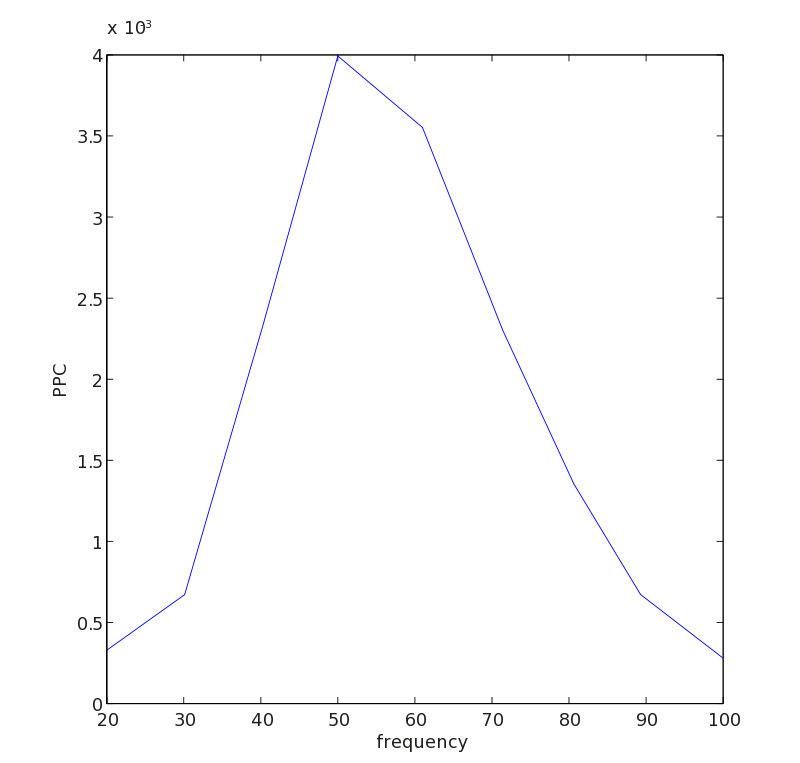It is often desired to study the evolution of the spike-LFP phase consistency over time. To do so, we run

``````param = 'ppc0'; % set the desired parameter
for k = 1:length(stsConvol.label)
cfg                = [];
cfg.method         = param;
excludeChan        = str2num(stsConvol.label{k}(6)); % this gives us the electrode number of the unit
chan = true(1,4);
chan(excludeChan)  = false;
cfg.spikechannel   = stsConvol.label{k};
cfg.channel        = stsConvol.lfplabel(chan);
cfg.avgoverchan    = 'unweighted';
cfg.winstepsize    = 0.05; % step size of the window that we slide over time
cfg.timwin         = 0.5; % duration of sliding window
statSts            = ft_spiketriggeredspectrum_stat(cfg,stsConvol);

statSts.(param) = permute(conv2(squeeze(statSts.(param)), ones(1,20)./20, 'same'),[3 1 2]); % apply some smoothing over 0.2 sec

figure
cfg            = [];
cfg.parameter  = param;
cfg.refchannel = statSts.labelcmb{1,1};
cfg.channel    = statSts.labelcmb{1,2};
cfg.xlim       = [-1 2];
ft_singleplotTFR(cfg, statSts)
end
``````

For example, the PPC TFR for unit ‘sig002a_wf’ reveals a specific increase in gamma-band synchronization after stimulus onset, increasing over time, as reported in Fries et al. (2008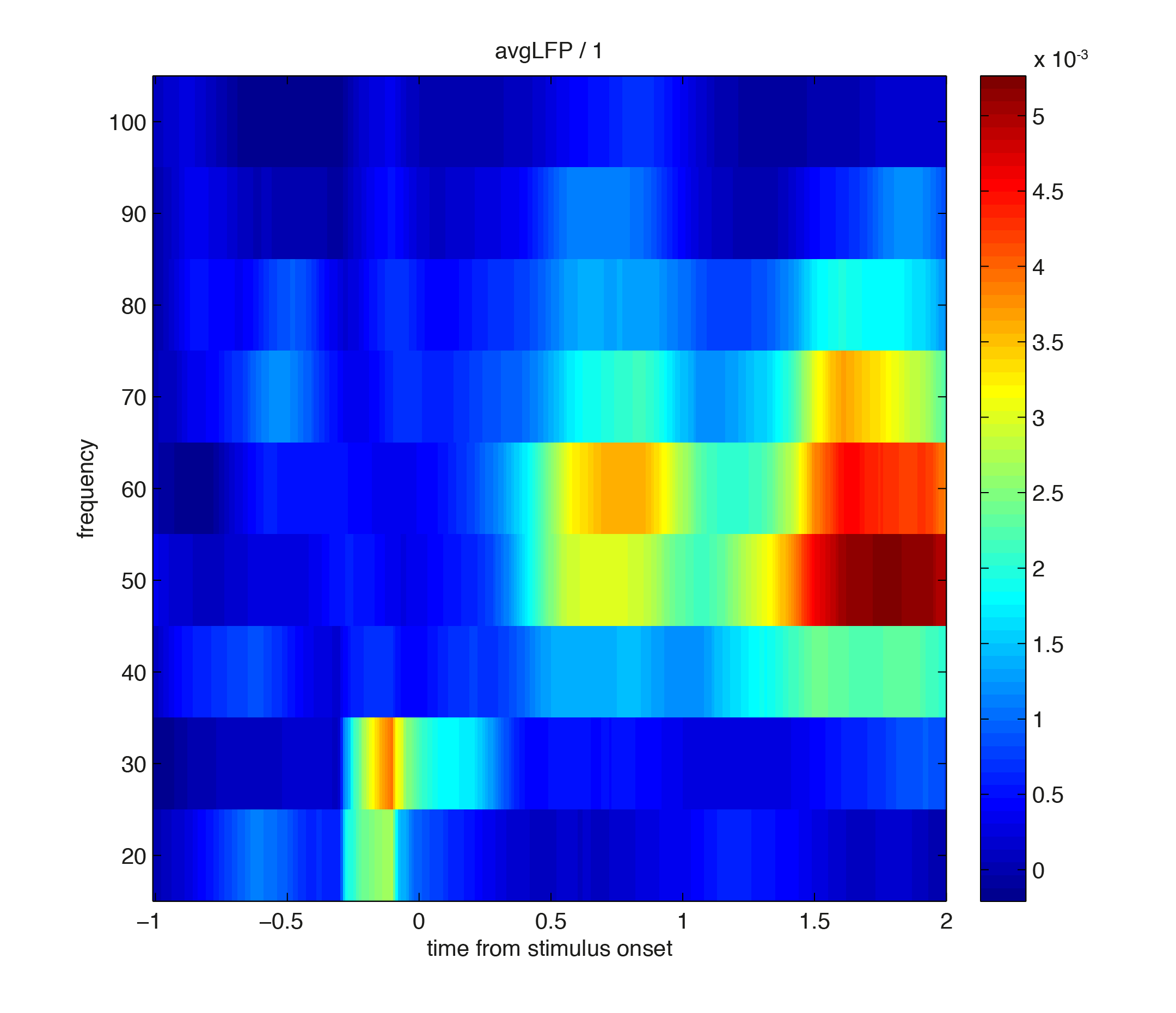Running the same script but now replacing param = ‘ppc0’ with ‘param = plv’ gives the following figur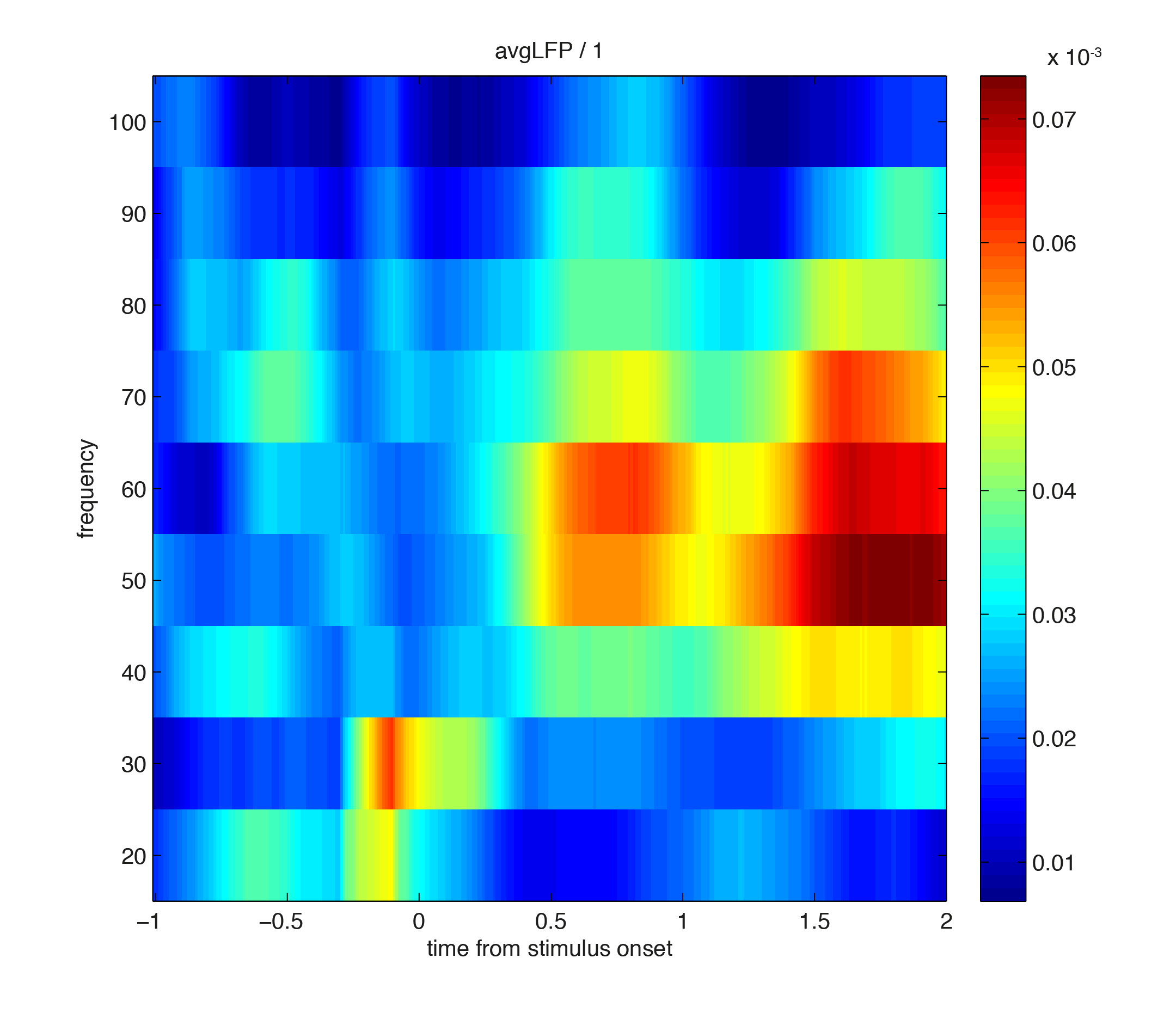Note that the ‘plv’ measure is (positively) biased by the number of spikes, and hence gives a less sharp contrast as pre-stimulus PLV values are biased upwards.

The output from ft_spiketriggeredspectrum_stat is a structure with the following content

``````statSts =
time: [1x155 double]
ppc0: [1x9x155 double] % can be plv, ang, ppc1, ppc2, ral
nspikes: [1x9x155 double]
labelcmb: {'sig003a_wf'  'avgLFP'}
freq: [20 30.1205 40.3226 50 60.9756 71.4286 80.6452 89.2857 100]
dimord: 'chancmb_freq_time'
cfg: [1x1 struct]
``````

In statSts.labelcmb all the combinations between the unit and the different LFPs are specified, for 9 frequencies and 155 time-points.

## Summary

We have shown how to compute measures of spike-LFP phase-coupling using the spike toolbox in FieldTrip. Time-frequency representations of mean spike-LFP phase or the spike-LFP phase consistency can be obtained. We have shown examples of how to implement measures of spike-LFP phase consistency that are not affected by the number of available spikes.

Further development of the functionality will be in the direction of computing inferential statistics on the phase consistency measures using permutation statistics.

You might want to continue with the spike tutorial, which presents more detailed analysis methods that are specific to the spikes.